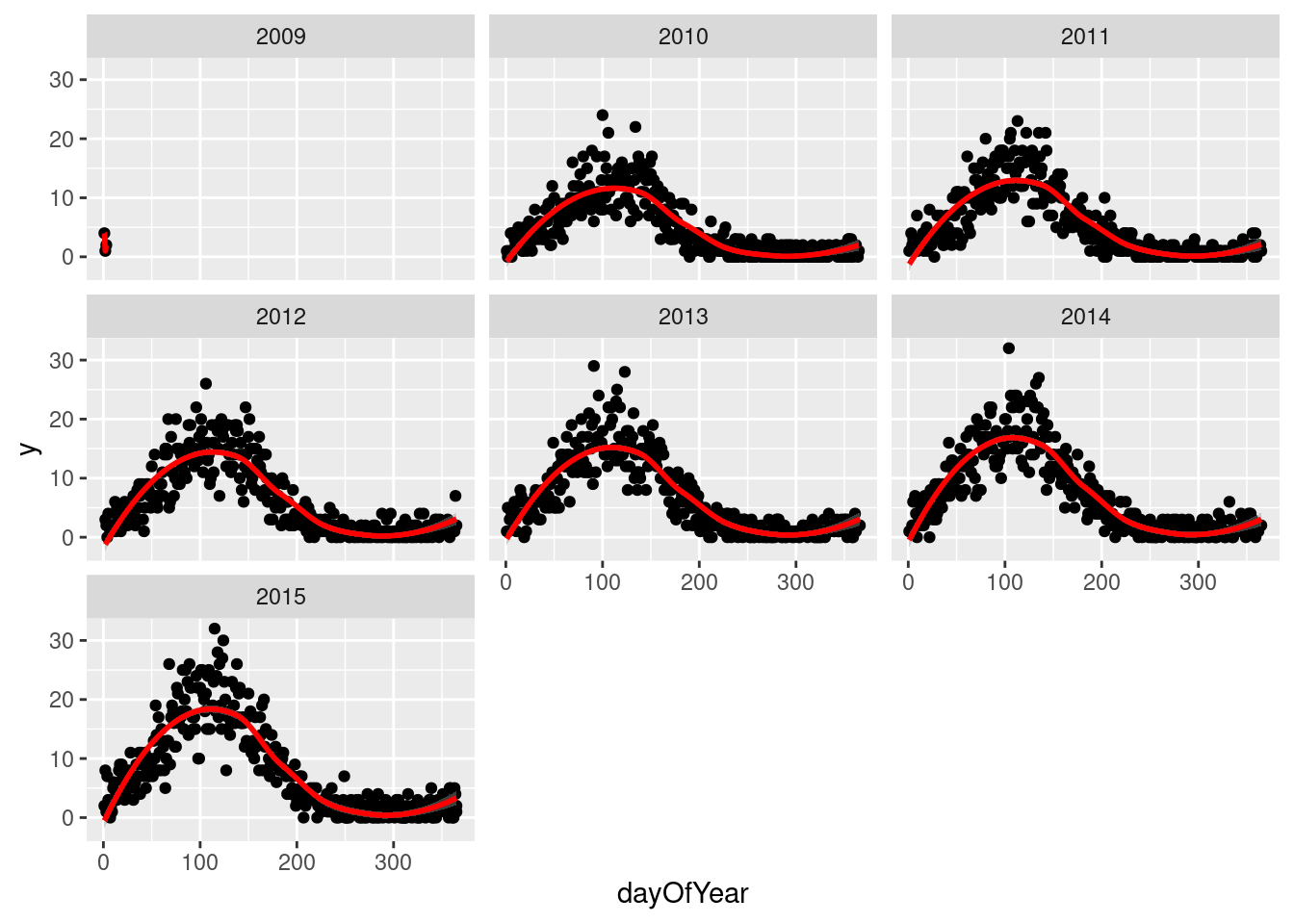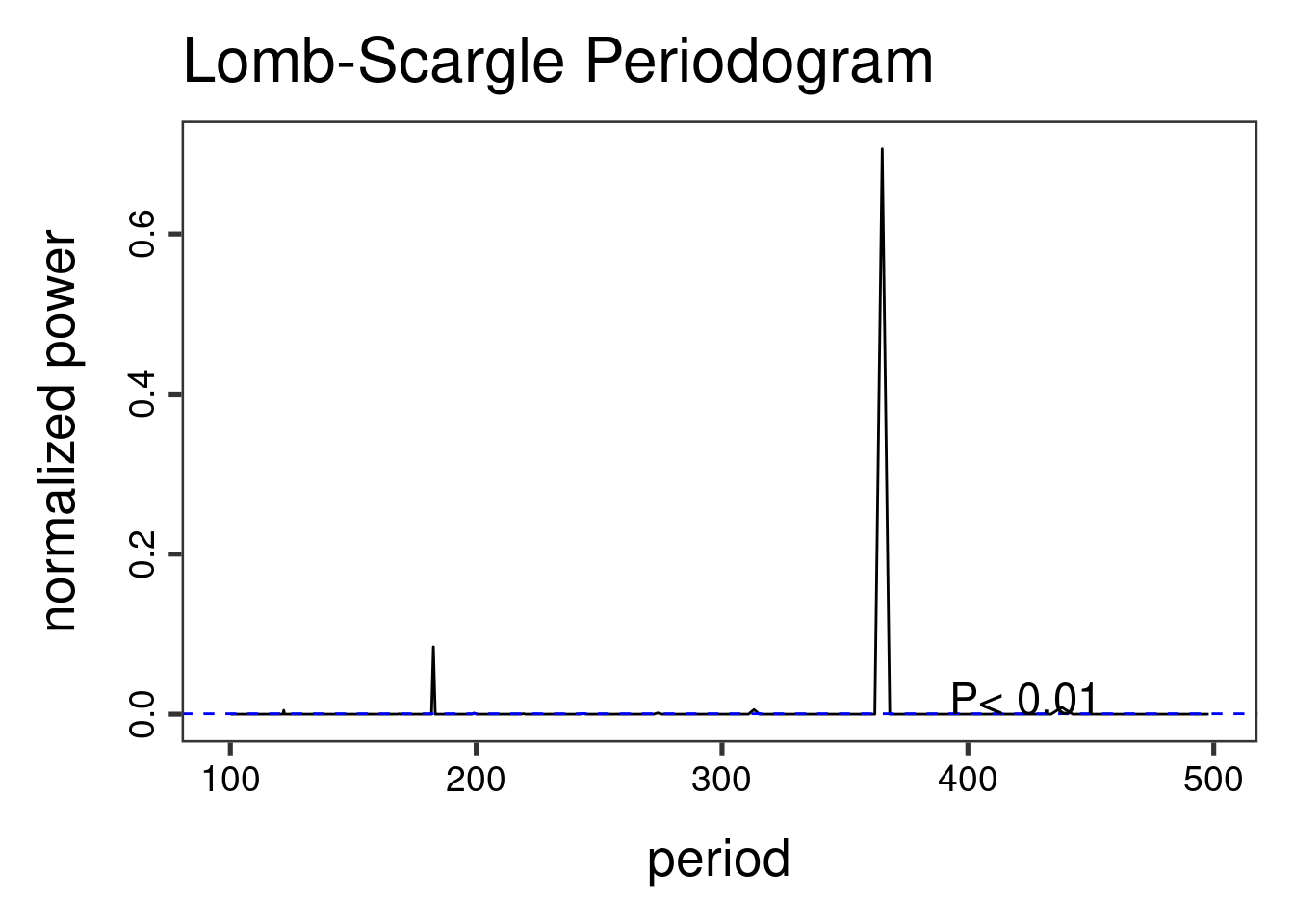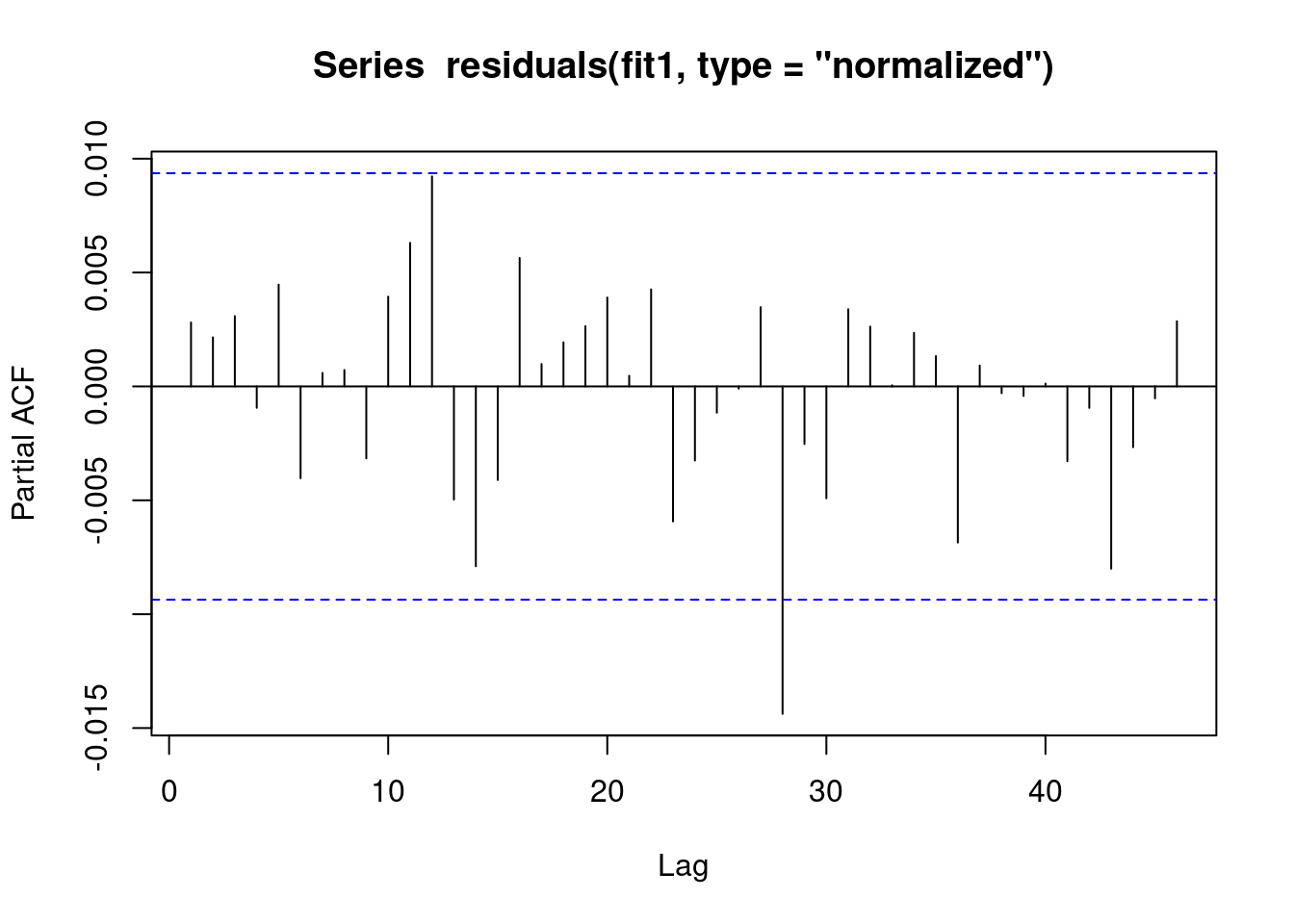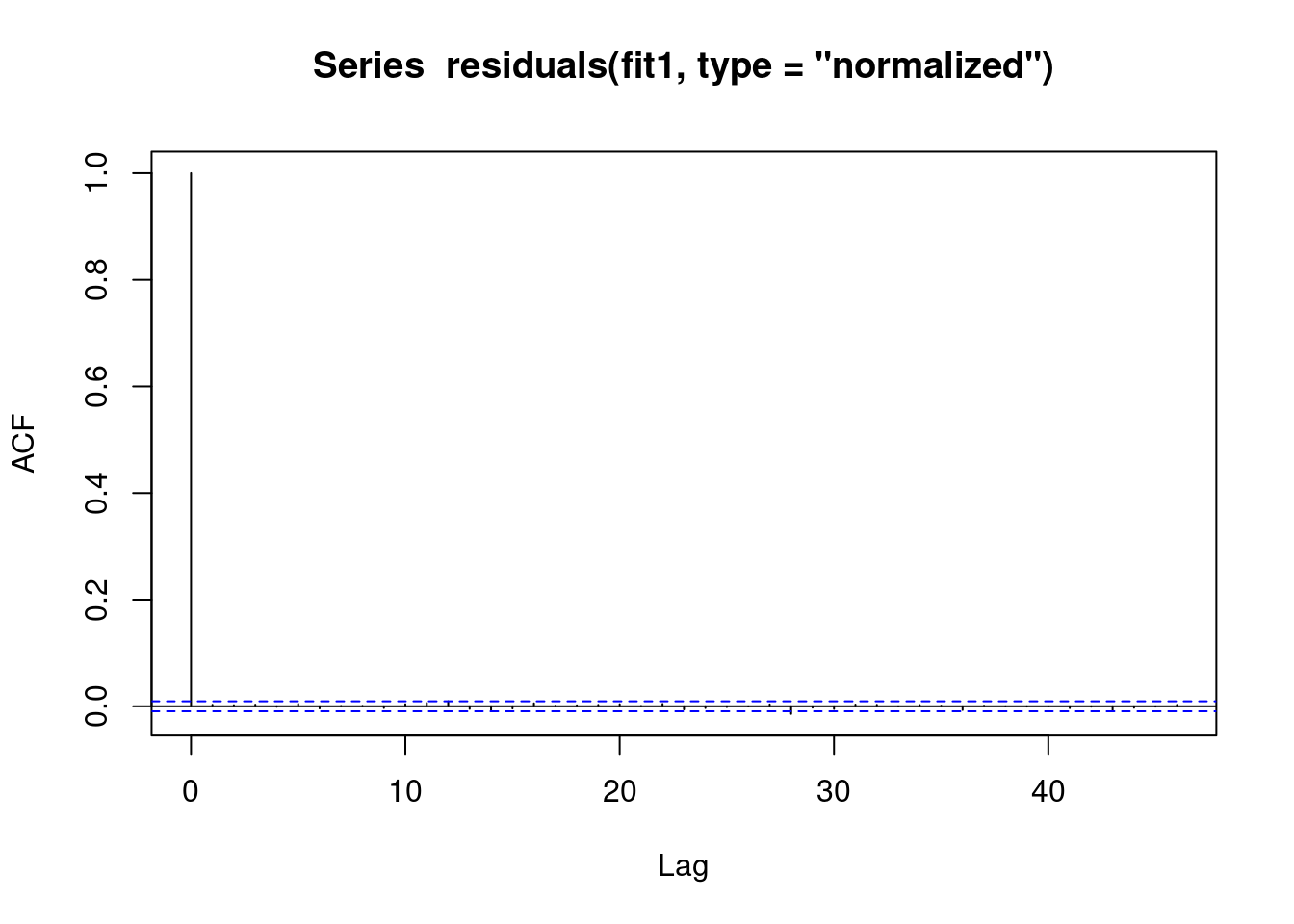## 5.1 Aim

We are given a dataset containing daily counts of diseases from multiple geographical areas. We want to identify:

• Does seasonality exist?
• If seasonality exists, when are the high/low seasons?
• Is there a general yearly trend (i.e. increasing or decreasing from year to year?)

## 5.2 Creating the data

The data for this chapter is available at: https://www.csids.no/longitudinal-analysis-for-surveillance/data/chapter_5.csv

``````library(data.table)
library(ggplot2)
set.seed(4)

AMPLITUDE <- 1.5
SEASONAL_HORIZONTAL_SHIFT <- 20

fylkeIntercepts <- data.table(fylke=1:20,fylkeIntercepts=rnorm(20))

d <- data.table(date=seq.Date(
from=as.Date("2010-01-01"),
to=as.Date("2015-12-31"),
by=1))
d[,year:=as.numeric(format.Date(date,"%G"))]
d[,week:=as.numeric(format.Date(date,"%V"))]
d[,month:=as.numeric(format.Date(date,"%m"))]

temp <- vector("list",length=20)
for(i in 1:20){
temp[[i]] <- copy(d)
temp[[i]][,fylke:=i]
}
d <- rbindlist(temp)

d[,yearMinus2000:=year-2000]
d[,dayOfSeries:=1:.N]

d[,dayOfYear:=as.numeric(format.Date(date,"%j"))]
d[,seasonalEffect:=sin(2*pi*(dayOfYear-SEASONAL_HORIZONTAL_SHIFT)/365)]
d[,mu := exp(0.1 + yearMinus2000*0.1 + seasonalEffect*AMPLITUDE)]
d[,y:=rpois(.N,mu)]``````

## 5.3 Investigation

We then drill down into a few years for fylke 1, and see a clear seasonal trend

``````q <- ggplot(d[fylke==1],aes(x=dayOfYear,y=y))
q <- q + facet_wrap(~year)
q <- q + geom_point()
q <- q + stat_smooth(colour="red")
q``````
```geom_smooth()` using method = 'loess' and formula 'y ~ x'``The Lomb-Scargle Periodogram shows a clear seasonality with a period of 365 days

``````// STATA CODE STARTS
insheet using "chapter_6.csv", clear

sort fylke date
by fylke: gen time=_n
tsset fylke time, daily

wntestb y if fylke==1

cumsp y if fylke==1, gen(cumulative_spec_dist)
by fylke: gen period=_N/_n

browse cumulative_spec_dist period
// STATA CODE ENDS``````
``````# RCODE
lomb::lsp(d\$y,from=100,to=500,ofac=1,type="period")``````## 5.4 Regression

First we create an `id` variable. This generally corresponds to geographical locations, or people. In this case, we only have one geographical location, so our `id` for all observations is `1`. This lets the computer know that all data belongs to the same group.

When we have panel data with multiple areas, we use the `MASS::glmPQL` function in R and the `meglm` function in STATA. In R we identify the geographical areas with `random = ~ 1 | fylke` and in STATA with `|| fylke:`.

``````// STATA CODE STARTS
gen cos365=cos(dayofyear*2*_pi/365)
gen sin365=sin(dayofyear*2*_pi/365)

meglm y yearminus2000 || fylke:, family(poisson) iter(10)
estimates store m1
meglm y yearminus2000 cos365 sin365 || fylke:, family(poisson) iter(10)
estimates store m2

predict resid, anscombe

lrtest m1 m2
// STATA CODE ENDS``````
``````# R CODE
d[,cos365:=cos(dayOfYear*2*pi/365)]
d[,sin365:=sin(dayOfYear*2*pi/365)]
fit0 <- MASS::glmmPQL(y~yearMinus2000, random = ~ 1 | fylke,
family = poisson, data = d)``````
``iteration 1``
``````fit1 <- MASS::glmmPQL(y~yearMinus2000 + sin365 + cos365, random = ~ 1 | fylke,
family = poisson, data = d)``````
``iteration 1``
``print(lmtest::lrtest(fit0, fit1))``
``````Likelihood ratio test

Model 1: y ~ yearMinus2000
Model 2: y ~ yearMinus2000 + sin365 + cos365
#Df LogLik Df Chisq Pr(>Chisq)
1   4
2   6         2                 ``````

We see that the likelihood ratio test for `sin365` and `cos365` was significant, meaning that there is significant seasonality with a 365 day periodicity in our data (which we already strongly suspected due to the periodogram).

We can now run/look at the results of our main regression.

``print(summary(fit1))``
``````Linear mixed-effects model fit by maximum likelihood
Data: d
AIC BIC logLik
NA  NA     NA

Random effects:
Formula: ~1 | fylke
(Intercept)  Residual
StdDev: 1.583744e-05 0.9976713

Variance function:
Structure: fixed weights
Formula: ~invwt
Fixed effects:  y ~ yearMinus2000 + sin365 + cos365
Value   Std.Error    DF   t-value p-value
(Intercept)    0.1122536 0.014488403 43797    7.7478       0
yearMinus2000  0.0989047 0.001109477 43797   89.1453       0
sin365         1.4095095 0.003695341 43797  381.4288       0
cos365        -0.5109375 0.003083683 43797 -165.6907       0
Correlation:
(Intr) yM2000 sin365
yearMinus2000 -0.979
sin365        -0.150  0.000
cos365         0.065 -0.001 -0.151

Standardized Within-Group Residuals:
Min          Q1         Med          Q3         Max
-3.19682240 -0.82387498 -0.07501834  0.63400484  5.82452468

Number of Observations: 43820
Number of Groups: 20 ``````

## 5.5 Residual analysis

We see that there is no evidence of autoregression in the residuals

``````// STATA CODE STARTS
pac resid if fylke==1
// STATA CODE ENDS``````
``````# R CODE
pacf(residuals(fit1, type = "normalized")) # this is for AR``````We see that there is no evidence of autoregression in the residuals

``````// STATA CODE STARTS
ac resid if fylke==1
// STATA CODE ENDS``````
``````# R CODE
acf(residuals(fit1, type = "normalized")) # this is for MA``````We also obtain the same estimates that we did in the last chapter.

``````b1 <- 1.4007640 # sin coefficient
b2 <- -0.5234863 # cos coefficient
amplitude <- sqrt(b1^2 + b2^2)
p <- atan(b1/b2) * 365/2/pi
if (p > 0) {
peak <- p
trough <- p + 365/2
} else {
peak <- p + 365/2
trough <- p + 365
}
if (b1 < 0) {
g <- peak
peak <- trough
trough <- g
}
print(sprintf("amplitude is estimated as %s, peak is estimated as %s, trough is estimated as %s",round(amplitude,2),round(peak),round(trough)))``````
`` "amplitude is estimated as 1.5, peak is estimated as 112, trough is estimated as 295"``
``print(sprintf("true values are: amplitude: %s, peak: %s, trough: %s",round(AMPLITUDE,2),round(365/4+SEASONAL_HORIZONTAL_SHIFT),round(3*365/4+SEASONAL_HORIZONTAL_SHIFT)))``
`` "true values are: amplitude: 1.5, peak: 111, trough: 294"``